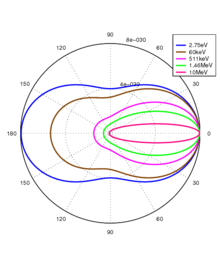# Horrible multiple TGraphPolar

Hi guys.
I do not understand Why I’m getting this horrible plot

It should be something like thisHere It is the code

``````#include "TGraphPolar.h"
#include "inc/RootImprove.h"
#include "inc/NuclearFunctions.h"

int main(int argc, char** argv ){
RootImprove R;
TCanvas *c2 = new TCanvas ("c2","",800,800);
c2->cd();

std::vector<TGraphPolar*> vgP;

const double e[]={2., 10., 50, 170., 511.}; //keV
int npoints = 100 ;
double min=0.;
double max=2.*TMath::Pi();
double r[npoints];
double theta[npoints];

TGraphPolar *gPaux;

for (int i = 0; i < npoints; i++)
theta[i]= min+i*(max-min)/npoints;

for (int k = 0; k < 5; k++) {
for (int i = 0; i < npoints; i++){
r[i] = KleinNishinaDC(theta[i], e[k] );
// std::cout <<r[i] <<" "<< theta[i]<<std::endl;
}
gPaux = new TGraphPolar(npoints,theta,r);
gPaux -> SetMarkerStyle(10);
gPaux -> SetMarkerSize(0.3);
gPaux -> SetLineColor(R.Color(k));
gPaux -> SetMarkerColor( R.Color(k) );
vgP.push_back( gPaux );

}
for (int k=0;k<5;k++)
vgP[k]->Draw("CP SAME");

c2->Update();
vgP -> GetPolargram()->SetTextColor(8);
vgP -> GetPolargram()->SetNdivPolar(0);

c2->Print("plot.jpg");
return 0;
}

``````

Any idea, what I am doing wrong
Regards

I am missing the two include files.
Can you provide a script we can run ?

sorry, here they areRootImprove.cpp (11.4 KB)
RootImprove.h (4.1 KB)
NuclearFunctions.h (347 Bytes)

It is just a matter of the axis range. Your radial axis goes between 40 and 80, but actually your values of r go below 40, so they jump to the negative side.

Just call:
`vgP -> GetPolargram()->SetRangeRadial(0,100);`

By the way, you may be interested in this code attached here, which does something similar to what you want, i.e. the Klein-Nishina cross section and Compton edge analysis, but in normal histograms. Should not be a big deal to convert to polar graphs. See http://nbn-resolving.de/urn:nbn:de:bsz:14-qucosa-204988 for details on the notation.

klein_nishina.cpp (16.2 KB)

That it is!!
Thank you very much ferhue.
By the way, I do not understand the ways how vgP -> GetPolargram()->SetNdivRadial(10); works according to the doxygen

``````Set the number of radial divisions: enter a number ij with 0<i<99 and 0<j<99.

i sets the major radial divisions.
j sets the minor radial divisions.
``````

So that I was expecting 1 major division and no minor divisions.
But I am not getting this.

The documentation is not very clear at this point. It should rather say:
Enter a number N = i*100 + j, where i (0-99) are the major radial divisions and j (0-99) are the minor ones. So j should occupy always 2 digits.

You may want to try 100 instead of 10.

Again ferhue thank you. That is the solution.

I know this should be in another topic, but in any case.
Do you know how to import UTF8 in titles. I need to use some Spanish accents (tildes) but
from all the available accents here
https://root.cern.ch/doc/master/classTLatex.html
the most similar is #acute, again this can be esthetics, but the way those accents looks are not so good. Even in the same example they provide you can realize it.

Hola,

yes, using #acute seems to be the easiest way. If you need better accents, I suggest that you save the canvas as .tex. Then, you compile with latex/tikz/pgf, where UTF8 is supported without problems.

Another way might be to use the Qt/Root plugin. See for example:

Note that a JIRA ticket is opened about the UTF-8 support.

This topic was automatically closed 14 days after the last reply. New replies are no longer allowed.